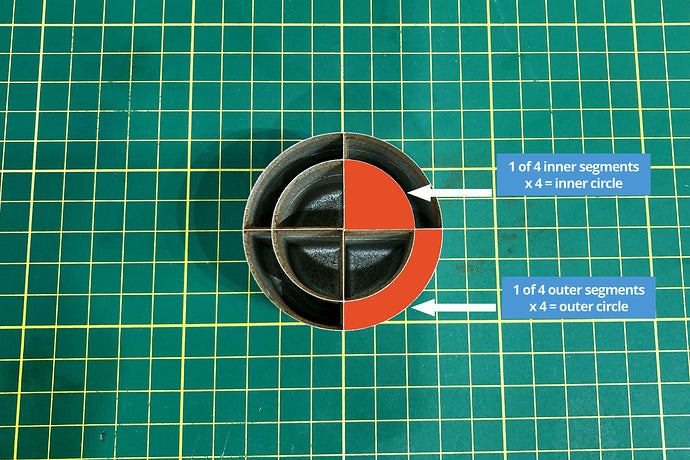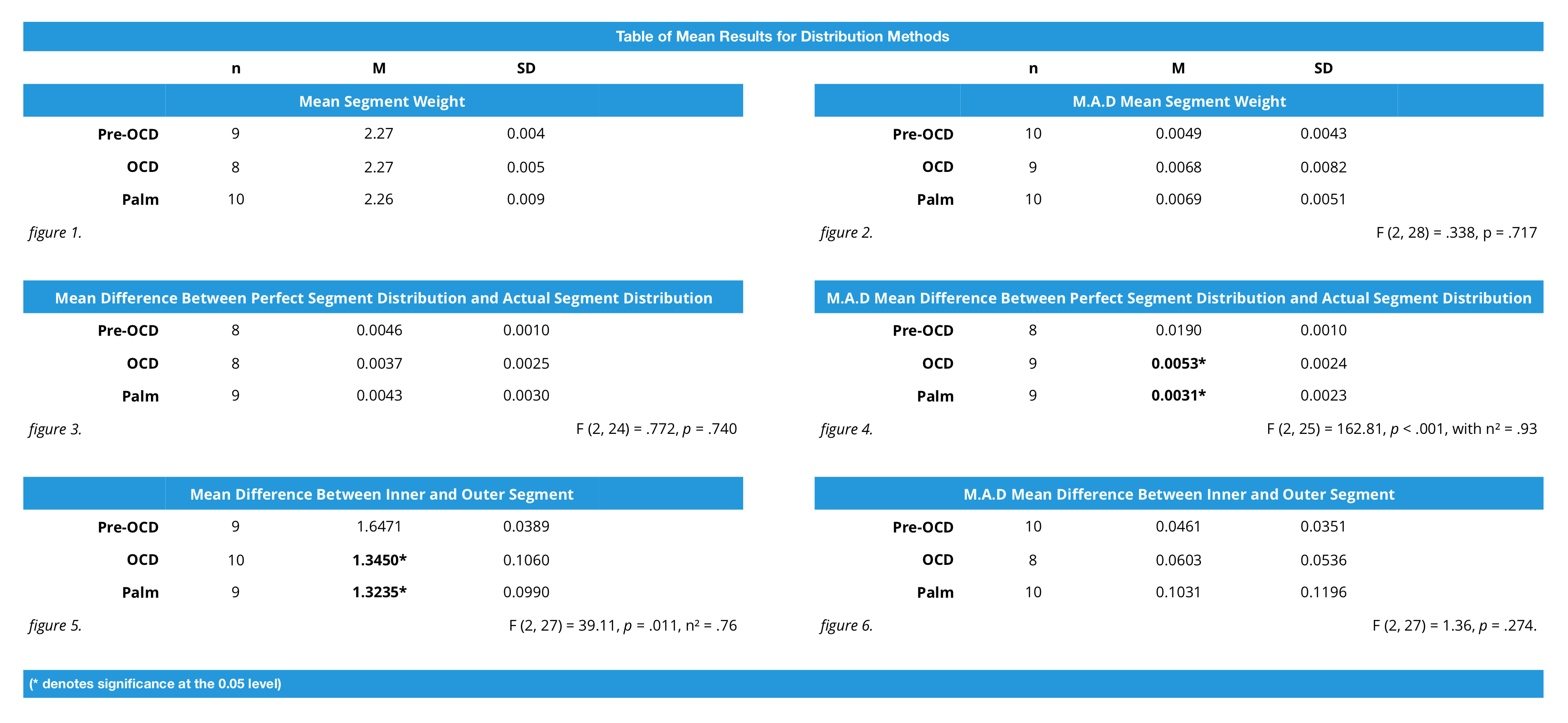# Published: Jan 25, 2018

The Great Distribution Debate: Results

Tldr: we found a statistical difference between using the OCD and finger distribution, and between palm tapping and finger distribution — but no significant difference between palm tapping and OCD. But we’re going to continue researching and have some cool ideas for the next experiment.

The longer version? Let’s start In June of last year, where we wanted to answer what we felt was a fairly simple question: “do distribution tools distribute?”

To test this, we manufactured a tool to insert into a 58mm VST basket filled with coffee (ground on a Mazzer grinder on a typical espresso setting) dividing the coffee “where it lay” into segments inside the tool. Though the inner and outer segments were shaped differently, they were of an equal volume. The tool could then be tipped over, and with a custom lid we could remove each individual section, weighing them using analytical scales accurate to 1/10,000th (!!!) of a gram. We have more on this tool and our initial ideas for this experiment here.A distribution tool that distributes effectively should, in principle, distribute an even amount of coffee into each segment.

We used this tool on three different styles of distribution: finger distribution the OCD (both following the protocol advised by Sasa Sastic) and palm tapping. 10 samples of each method were taken.

Originally we planned on 20 samples each, but this was a little ambitious. Each sample produced 10 data points: the dose itself, wastage between the outside of the tool and the curve of the basket, and the eight segments themselves. This culminated in 300 measurements taken over two days. These results were then statistically analysed in SPSS, a statistical analysis tool designed by IBM.

We had to organise the data in a meaningful way for analysis, so we first averaged the segment measurements of each sample, condensing them into one number. This resulted in an average segment size of the 10 measurements for each distribution method. Figure 1, in turn, shows the average for those numbers.These are descriptive statistics only, as we had nothing to meaningfully compare them to individually. However, we could compare the segment average for each distribution method to the total average shown in figure 1, giving us what’s known as the “mean absolute deviation” (MAD) which we then average again. This is a good measure for dispersion of the data, with a lower number showing less dispersion, therefore better or even distribution of each segment. Figure 2 shows these results.

Our initial analysis showed these results were normally distributed, and outliers were removed. While this affected the assumption of equal sample sizes, the analysis of variance we used (ANOVA) is considered fairly robust for moderate departures from this assumption. We could then continue with a one-way ANOVA, a statistical analysis of variance. Levene’s Test, a measure testing if the variances in the sample population are equal, was not significant, so we could also assume equality of variance.

This one-way ANOVA was not significant. This was an inconclusive measurement in trying to discern which distribution method from the experiment was most effective. Boo.

But! We gained a wealth of data from this experiment, so we could try a different angle.

As we recorded the total dose for each sample, along with the wastage, we could subtract the wastage from the dose, and divide the result by eight, giving us a proxy for what could be considered “perfect distribution” for each segment, for each sample. We then subtract this number from the average segment size for each corresponding sample, giving us the difference between “perfect distribution” and “actual distribution”. Converting these numbers to absolute values, we could say the lower this number, the better the distribution. Figure 3 shows these results.

The results were relatively even, with the OCD again slightly bumping ahead of the pack with a lower segment average. However, another one-way ANOVA for these results again was not significant.

So we calculated the MAD of the mean difference between perfect distribution and actual distribution, ran this through another one-way ANOVA (figure 4) and success! We had a significant result! The analysis showed 93% of the variance could be explained by distribution method … Which means there’s virtually no difference between distribution methods, in the sense they all vary.

But! Post Hoc analysis of the results showed significant results were confined to post-OCD and palm tapping methods. Using the MAD mean difference between perfect distribution and actual distribution, this showed using an OCD after finger distribution brought you closer to perfect distribution. It also showed using palm tapping instead of finger distribution also brought you closer to perfect distribution. It did not show using the OCD over palm tapping was better, or that palm tapping over the OCD was better. In this comparison, there was no significant difference between the two.

Hmmm. We needed to dive deeper, so there was one final angle we could take. As we had measurements for the outer segment of each sample, and measurements for the inner segment of each sample, we could argue an effective distribution method would have little to no difference between the inner and outer segment measurements. Averaging these numbers for each sample would give us a figure where a lower number would mean better or even distribution. Figure 5 shows these results.

We had success again! Another one-way ANOVA showed a significant difference between the means. This time 76% of the variance could be explained by distribution method. But! Post Hoc analysis again showed this difference was between pre-OCD and OCD, and pre-OCD and palm tapping — again not between OCD and palm tapping. Here palm tapping had a lower mean difference (as well as a lower variance) but this two 1/100ths of a gram difference was not statistically significant. Another one-way ANOVA of the M.A.D mean difference between inner and outer segment (figure 6) was equally insignificant.

There were a few limitations to this experiment that need mentioning. Obviously, we’d have preferred a larger sample size. However, the design of this experiment did not lend itself towards easy sampling. In this sense, it may be more practical to design an experiment focusing on the same segmentation of the puck, but beneath the basket as the shot is actually pouring. The idea of “perfect distribution” meaning equal segment sizes would remain with this design. There’s also the practical variable of tamping that was absent from this experiment. It seems common sense downward pressure on the puck would affect distribution. Both these considerations we hope to focus on in the future.

So — a neat summary of these results? 300+ measurements and six ANOVA’s later, we found a statistically significant difference between using the OCD or palm tapping over finger distribution. However, the OCD is no more an effective form of distribution than palm tapping. Based on this though, we’re going to continue researching and experimenting to find a conclusive answer to this question: “do distribution tools distribute?”